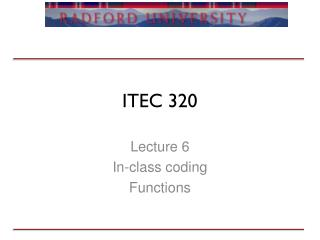DownloadDownload PresentationITEC 320

# ITEC 320

Télécharger la présentation## ITEC 320

- - - - - - - - - - - - - - - - - - - - - - - - - - - E N D - - - - - - - - - - - - - - - - - - - - - - - - - - -
##### Presentation Transcript

1. ITEC 320 Lecture 6 In-class coding Functions

2. Review • Types • Homework • Website is up • Questions?

3. VIM • Dr. Okie has been providing VIM information • Do you want a VI tutorial? • What are the advantages of knowing a power editor?

4. Outline • Procedures • Functions • Problems

5. Functions • Similar to java methods with a non-void return type • Must have a return type • Must be defined before use • Cannot modify parameters

6. Syntax function sumArray(a: Int_Array) return integer is s: Integer := 0; -- holds the sum begin for i in a'range loop s := s + a(i); end loop; return s; end sumArray;

7. Procedures • No return type allowed • Parameters can be changed • In (default) • Out (allows passing back to the caller) • In Out (pass in and out) • What about the get procedure’s parameter? • Structure charts help

8. Syntax with ada.text_io; use ada.text_io; with ada.integer_text_io; use ada.integer_text_io; procedure inout is procedure vars(a: in Integer; b: in out Integer; c: out Integer) is begin b:= b+a; c:= b+3; end vars; x: Integer; y: Integer; z: Integer; begin x:=2; y:=3; vars(x,y,z); put(x'img & "|" & y'img & "|" & z'img); end inout;

9. Issues • Assigning to an in parameter • Using an out parameter’s values in the procedure (by default it can be anything) • Forgetting to assign a value to an out parameter • Can’t fool variables • I.e. passing an in mode variable to another procedure where it is an out mode parameter

10. Discussion • Web example

11. Problems • Write a function/procedure that returns whether or not a String is a palindrome

12. Problems • Given n of 1 or more, return the factorial of n, which is n * (n-1) * (n-2) ... 1. Compute the result recursively (without loops).

13. Summary • Functions • Procedures